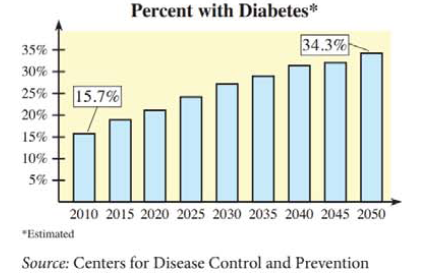Chapter 9.6, Problem 56E### Mathematical Applications for the ...

11th Edition
Ronald J. Harshbarger + 1 other
ISBN: 9781305108042

#### Solutions

Chapter
Section### Mathematical Applications for the ...

11th Edition
Ronald J. Harshbarger + 1 other
ISBN: 9781305108042
Textbook Problem

# Diabetes The figure shows the percent of the U.S. population with diabetes (diagnosed and undiagnosed) for selected years from 2010 and projections to 2050. Assume that this percent can be modeled by y = 6.97 ( 0.5 x + 5 ) 0.495 where y is the percent and x is the number of years past 2010.Source: Centers for Disease Control and Prevention(a) Use the figure to find the average rate of change from 2010 to 2050.(b) Use the model to find the instantaneous rate of change of the U.S. population with diabetes in 2020. Is the average rate found in part (a) a good approximation of the 2020 instantaneous rate?(c) Use the model to find and interpret the instantaneous rate of change of the percent of the U.S. population with diabetes in 2050.

(a)

To determine

To calculate: The average rate of change from 2010 to 2050. The figure shows the percent of the U.S. population with diabetes for selected years from 2010 and projections to 2050. Assume that this percent can be modeled by y=6.97(0.5x+5)0.495 where y is the percent and x is the number of years past 2010.

Explanation

Given Information:

The figure shows the percent of the U.S. population with diabetes,

Formula used:

The average rate of change of a function f(x) at two values a and b is given by f(x)=f(b)f(a)ba.

Calculation:

Consider the provided function,

y=6.97(0.5x+5)0.495

According to the graph,

In year 2010, the percentage was 15.7%, and in the year 2050 the percentage will be 34

(b)

To determine

To calculate: The instantaneous rate of change of the U.S. population with diabetes in 2020. Also, determine if the average rate of change from 2010 to 2050 a good approximation of the 2020 instantaneous rate. The figure shows the percent of the U.S. population with diabetes for selected years from 2010 and projections to 2050. Assume that this percent can be modeled by y=6.97(0.5x+5)0.495 where y is the percent and x is the number of years past 2010.

(c)

To determine

To calculate: The instantaneous rate of change of the percent of the U.S. population with diabetes in 2050. The figure shows the percent of the U.S. population with diabetes for selected years from 2010 and projections to 2050. Assume that this percent can be modeled by y=6.97(0.5x+5)0.495 where y is the percent and x is the number of years past 2010. Interpret the answer.

### Still sussing out bartleby?

Check out a sample textbook solution.

See a sample solution

#### The Solution to Your Study Problems

Bartleby provides explanations to thousands of textbook problems written by our experts, many with advanced degrees!

Get Started

#### Simplify the expressions in Exercises 97106. (xy)1/3(yx)1/3

Finite Mathematics and Applied Calculus (MindTap Course List)

#### In Exercises 2340, find the indicated limit. 25. limx3x

Applied Calculus for the Managerial, Life, and Social Sciences: A Brief Approach

#### Evaluate: 12x21xdx. a) 322 b) 2+21 c) 4+2 d) 14

Study Guide for Stewart's Single Variable Calculus: Early Transcendentals, 8th

#### For j – 2k 3i – 4k 3i + j – 2k 0 (zero vector)

Study Guide for Stewart's Multivariable Calculus, 8th